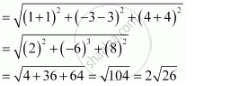# Find the Distance Between the Pairs of Points: (–1, 3, –4) and (1, –3, 4) - Mathematics

Find the distance between the pairs of points:

(–1, 3, –4) and (1, –3, 4)

#### Solution

The distance between points P(x1, y1, z1) and P(x2, y2, z2) is given by

PQ = sqrt((x_2 -x_1)^2 + (y_2-y^1)^2 + (z_2 - z_1)^2)

Distance between points (–1, 3, –4) and (1, –3, 4)Concept: Three - Dimensional Geometry - Distance Between Two Points
Is there an error in this question or solution?
Chapter 12: Introduction to Three Dimensional Geometry - Exercise 12.2 [Page 273]

#### APPEARS IN

NCERT Class 11 Mathematics
Chapter 12 Introduction to Three Dimensional Geometry
Exercise 12.2 | Q 1.3 | Page 273

Share